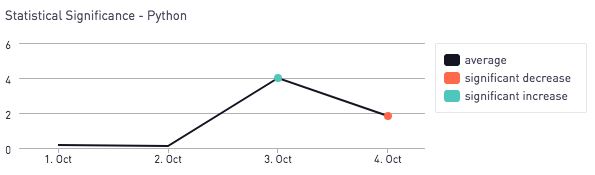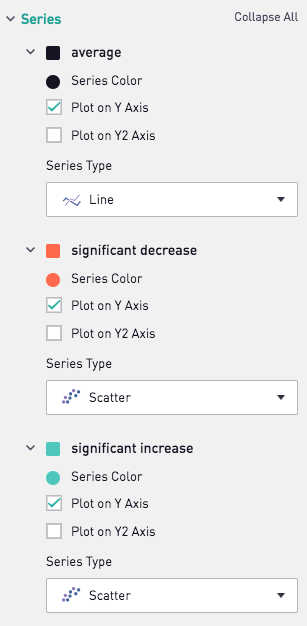Visualizing Statistical Significance in Samples over Time - PythonLet's say you're tracking a KPI, and want to see if any changes in your KPI are statistically significant. It would be nice to mark these points on your line graph to call attention to these particular increases and decreases. In the above visualization, we used Periscope's Python Integration to calculate statistical significance day by day for a generated data set

Our raw data frame has 2 columns:

• "day" - The day of the observation
• "value" - The value corresponding to the individual observation

We use the Python 3.6 code below to add a column for statistical significance, and also do some other minor transformations on the data frame to prep it for plotting via the Periscope visualization settings. Note that the default p-value threshold is 0.05, but this can be tailored in the final function call at the end of the code block below.

Note that the code below assumes  t-distribution and applies a two-tailed test. By default we also assume an independent t-test (samples are different between observations), but you can adjust the "method" parameter of the final function to use a "dependent" t test if that better describes your sample.

import numpy as np
import pandas as pd
from scipy import stats

# Generate data for example. Remove this section of code to analyze the SQL output
day = ['2018-10-01'] * 50 + ['2018-10-02'] * 50 + ['2018-10-03'] * 50 + ['2018-10-04'] * 50
val = np.random.normal(0, 1, 50).tolist() + np.random.normal(-0.05, 2, 50).tolist() + np.random.normal(4, 1, 50).tolist() + np.random.normal(2, 1, 50).tolist()
df = pd.DataFrame(
{'day': day,
'value': val,
})

# Functions: stat_sig, a function that outputs a dataframe containing a column, significance, that calls out any statistical significant changes between groups over time
# Inputs: a dataframe with raw data df, that contains at least 2 columns: one with the "day," and one with the "value." Default signifcance level is set to 0.95. Default methodology is an independent t-test
# Outputs: a dataframe containing a column sig that points out staistically significant changes
def stat_sig(df, interval = 0.95, method = 'independent'):

# Sort the data frame by the "day" column so the data is in chronilogical order
df = df.sort_values(by=['day'])

# Initiate the summary data frame
unique_x = df.day.unique()
first_x = unique_x
a0 = df.where(df.day == first_x).dropna()['value']
first_mean = np.mean(a0)
stat_sig_df = pd.DataFrame([[first_x, first_mean, 'average']],columns = ['day','mean','significance'])

# Loop through remaining rows and test for statistical significance
for x in range(unique_x.size - 1):
first_x = unique_x[x]
second_x = unique_x[x + 1]
a = df.where(df.day == first_x).dropna()['value']
b = df.where(df.day == second_x).dropna()['value']
mean_a = np.mean(a)
mean_b = np.mean(b)
if method == 'independent':
t, p = stats.ttest_ind(a,b)
else:
t, p = stats.ttest_rel(a,b)

if (p < (1 - interval)/2 and mean_a < mean_b):
change = 'significant increase'
elif (p < (1 - interval)/2 and mean_a > mean_b):
change = 'significant decrease'
else:
change = 'average'

stat_sig_df_add = pd.DataFrame([[second_x, mean_b, change]],columns = ['day','mean','significance'])
stat_sig_df = stat_sig_df.append(stat_sig_df_add, ignore_index=True)

# OPTIONAL: Comment out the below chunk if you would like to view the significance test results in a table
significant_results = stat_sig_df.where(stat_sig_df.significance != 'average').dropna()
significant_results.significance = 'average'
stat_sig_df = stat_sig_df.append(significant_results, ignore_index=True)

return stat_sig_df

# Pass through stat_sig(df, confidence level) into periscope.output to view the signficance test results. To plot these on a graph. Select "line chart" as your chart type, with sig as your series. Update the series types for "Significant Decrease" and "Significant Increase" to "Scatter"
periscope.output(stat_sig(df, method = 'independent'))

Finally we apply the following visualization settings. We apply red dots where there is a significant decrease in the KPI from the previous day to the next day, and a green dot if there is a significant increase.Prefer R? Check out the R equivalent of this post!